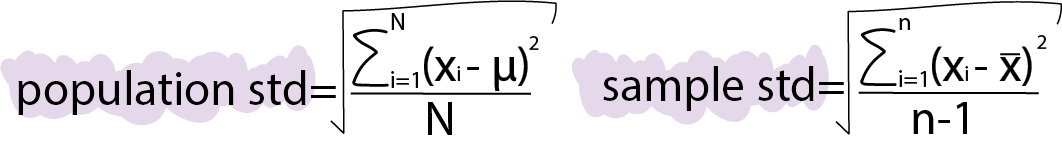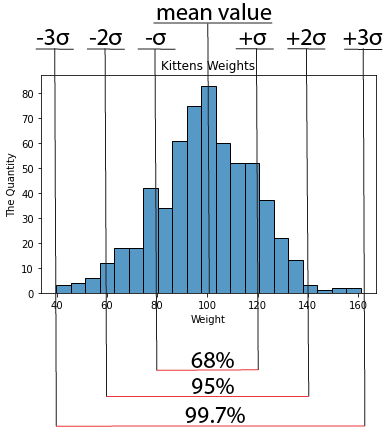Course Content

# Learning Statistics with Python

Learning Statistics with Python

##Standard deviation

The next term is standard deviation. This value is similar to variance because standard deviation is the square root of variance. Therefore, the formulas will differ for the population and sample.Definition

Standard deviation is a measure of how data is spread out in relation to the mean.

68–95–99.7 rule

If the population follows a Normal Distribution, we have the empirical 68-95-99.7 rule. According to this rule:

• Approximately 68% of the data falls within 1 standard deviation (σ) from the mean.
• About 95% of the data falls within 2σ of the mean.
• Nearly 99.7% of the data falls within 3σ of the mean.

When dealing with samples, the percentages might not be precisely accurate, but you can expect them to be quite close to the values in the rule, especially with larger sample sizes.

Example

To illustrate this, let's examine a sample of kitten weights measured in grams:Take a look at the illustration. In this scenario, we are working with the following data:

• Mean value = 100 grams.
• Standard deviation (represented by the sigma symbol in the picture) = 20 grams.

As mentioned earlier, one standard deviation above and below the mean encompasses 68% of the values. In this instance, those values range from mean - standard deviation = 100 - 20 = 80 to mean + standard deviation = 100 + 20 = 120.#### You are dealing with a normal data distribution with a mean value of 1500 and a standard deviation of 100. Now, let's associate the percentage of data with the corresponding numerical range.

68%
___

95%
___

99.7%
___

Click or drag`n`drop items and fill in the blanks[1400, 1600][1300, 1700][1200, 1800]Everything was clear?

Section 3. Chapter 4